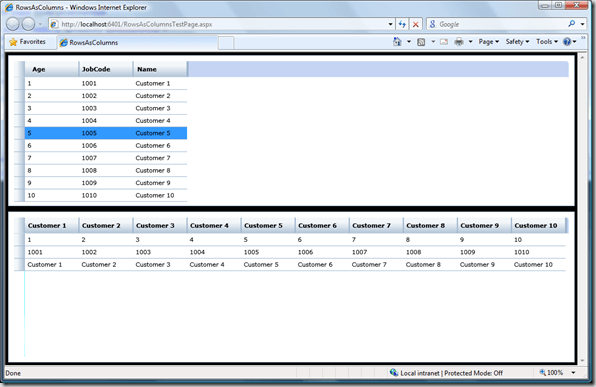A common scenario when displaying tabular data is to flip the axis so your rows becomes columns and vice versa. This can be easily achieved when you know the shape of your data. For example imagine we have a Customer class with 3 properties:  Name, Age and JobCode. Let’s say that we have 5 customers in our data repository. If we want to select just the names we can do something like this:

IList<Customer> customers = Customer.GetAll();

var names =

new

{

Customer1 = customers.Name,

Customer2 = customers.Name,

Customer3 = customers.Name,

Customer4 = customers.Name,

Customer5 = customers.Name

};

Imagine that another Customer is added and suddenly this code have to changed. Obviously this won’t scale even in a short period of time. So what is the solution?

Introducing Transpose<T> extension method

Transpose is an operation used in linear algebra to transpose (of course) a matrix. In our case Transpose is an extension method with the following signature:

public static IEnumerable Transpose<T>(this IEnumerable<T> source)

But what did Transpose do under the covers? Here are the recipe ingredients:

• 1 tablespoon of DynamicClass.
• 2 tablespoons of Reflection.
• 1 cup of Expression Trees.
• 1 slice of LINQ.
• 2 slices of functional programming.

Here is the code itself:

public static class EnumerableExtension

{

private static readonly MethodInfo GetValueMethod =

(from m in typeof(PropertyInfo).GetMethods()

where m.Name == "GetValue" && !m.IsAbstract

select m).First();

private static readonly ConstantExpression NullObjectArrayExpression =

Expression.Constant(null, typeof(object[]));

public static IEnumerable Transpose<T>(this IEnumerable<T> source)

{

if (source == null)

{

throw new ArgumentNullException("source");

}

return TransposeCore(source);

}

private static Delegate CreateSelectorFunc<T>(IEnumerable<T> source)

{

T[] list = source.ToArray();

DynamicProperty[] dynamicProperties =

list.Select(i => new DynamicProperty(i.ToString(), typeof(object))).ToArray();

Type transposedType = ClassFactory.Instance.GetDynamicClass(dynamicProperties);

ParameterExpression propParam = Expression.Parameter(typeof(PropertyInfo), "prop");

var bindings = new MemberBinding[list.Length];

for (int i = 0; i < list.Length; i++)

{

MethodCallExpression getter =

Expression.Call(

propParam,

GetValueMethod,

Expression.Constant(list[i]),

NullObjectArrayExpression

);

bindings[i] = Expression.Bind(transposedType.GetProperty(dynamicProperties[i].Name), getter);

}

LambdaExpression selector =

Expression.Lambda(

Expression.MemberInit(

Expression.New(transposedType),

bindings),

propParam);

return selector.Compile();

}

private static IEnumerable TransposeCore<T>(IEnumerable<T> source)

{

List<PropertyInfo> properties = typeof(T).GetProperties().ToList();

Delegate selector = CreateSelectorFunc(source);

foreach (PropertyInfo property in properties)

{

yield return selector.DynamicInvoke(property);

}

}

}

Note that some iterator best guidelines are also in place. And the final result is: